# Alcohol & Ether - JEE Advanced Previous Year Questions with Solutions

JEE Advanced Previous Year Questions of Chemistry with Solutions are available at eSaral. Practicing JEE Advanced Previous Year Papers Questions of Chemistry will help the JEE aspirants in realizing the question pattern as well as help in analyzing weak & strong areas. Simulator   Previous Years JEE Advance Questions
Q. Amongst the following, the total number of compounds soluble in aqueous NaOH is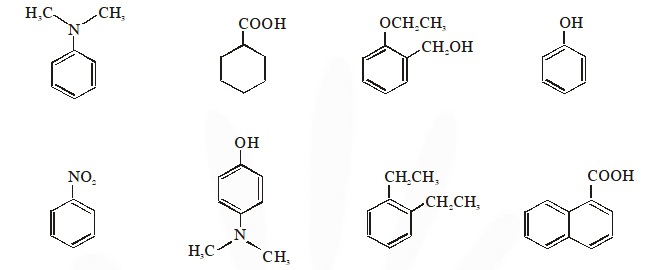JEE Advance 2010
Ans. (4)
Q. In the reaction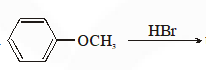the products are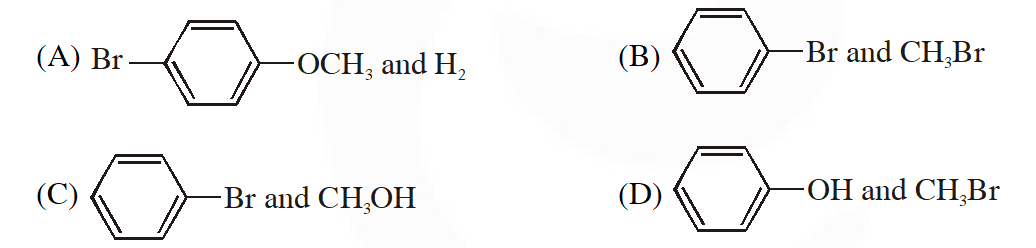JEE Advance 2010
Ans. (D)
Q. The major product in the following reaction is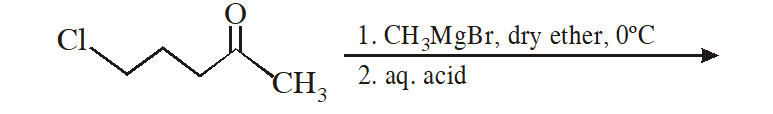(A)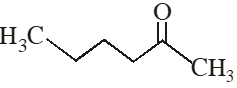(B)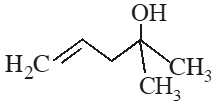(C)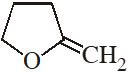(D)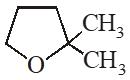JEE Advance 2014
Ans. (D)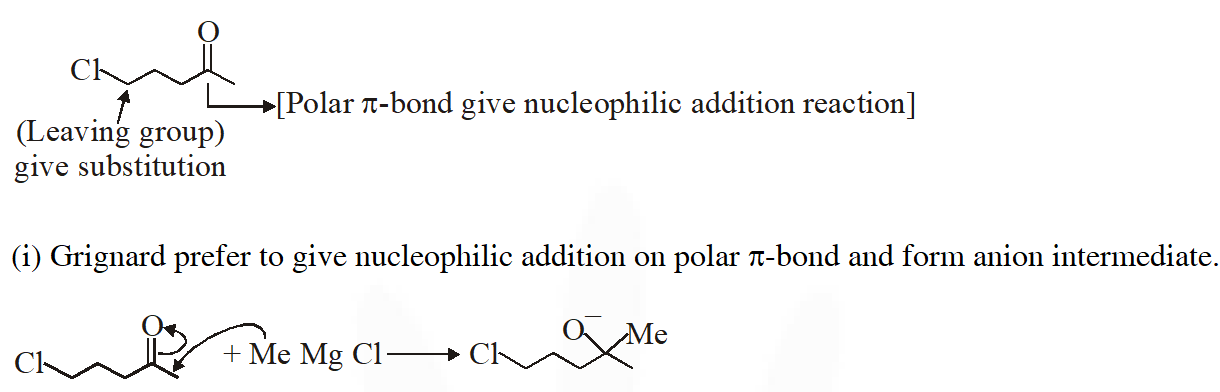(ii) In next step anion give intramolecular nucleophilic substitution reaction & form 5 membered ring.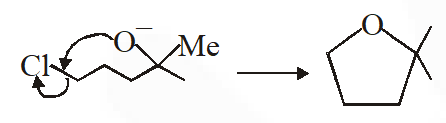Q. The acidic hydrolysis of ether (X) shown below is fastest when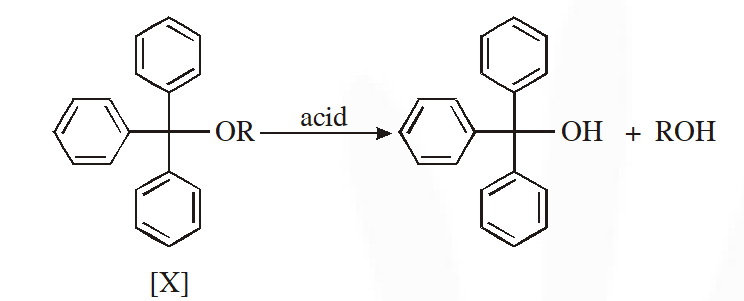(A) one phenyl group is replaced by a methyl group (B) one phenyl group is replaced by a para-methoxyphenyl group (C) two phenyl groups are replaced by two para-methoxyphenyl group (D) no structural change is made to X JEE Advance 2014
Ans. (C)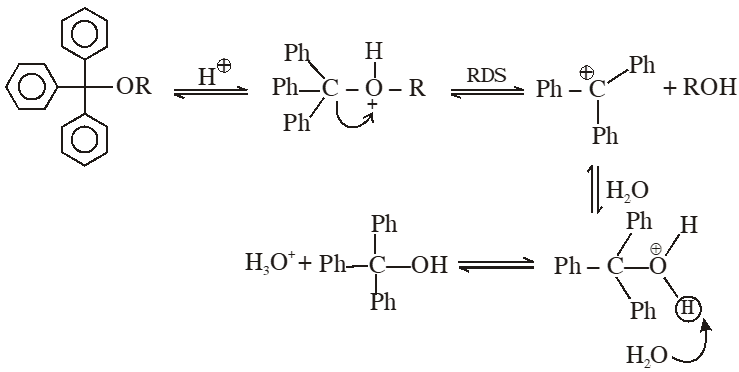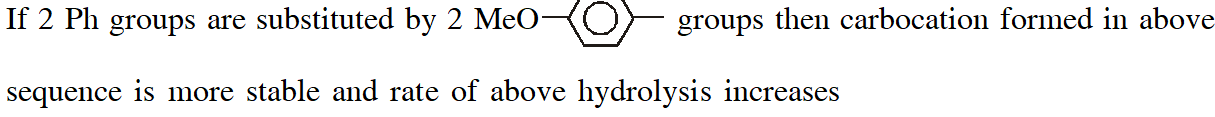Q. The correct combination of names for isomeric alcohols with molecular formula $\mathrm{C}_{4} \mathrm{H}_{10} \mathrm{O}$ is/are- (A) tert-butanol and 2-methylpropan-2-ol (B) tert-butanol and 1, 1-dimethylethan-1-ol (C) n-butanol and butan-1-ol (D) isobutyl alcohol and 2-methylpropan-1-ol JEE Advance 2014
Ans. (A,C,D) (A,C,D) The combination of names for isomeric alcohols with molecular formula $\mathrm{C}_{4} \mathrm{H}_{10} \mathrm{O}$ is/are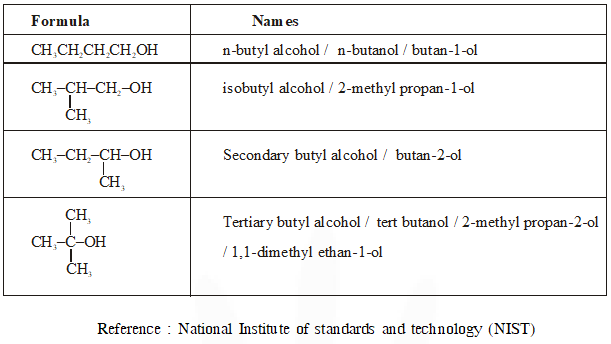Q. Consider all possible isomeric ketones including stereoisomers of MW = 100, All these isomers are independently reacted with $\mathrm{NaBH}_{4}$ (NOTE : stereoisomers are also reacted separately). The total number of ketones that give a racemic product(s) is/are JEE Advance 2014
Ans. 5
Q. The desired product X can be prepared by reacting the major product of the reactions in LIST-I with one or more appropriate reagents in LIST-II. (given, order of migratory aptitude: aryl > alkyl > hydrogen)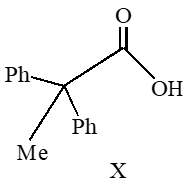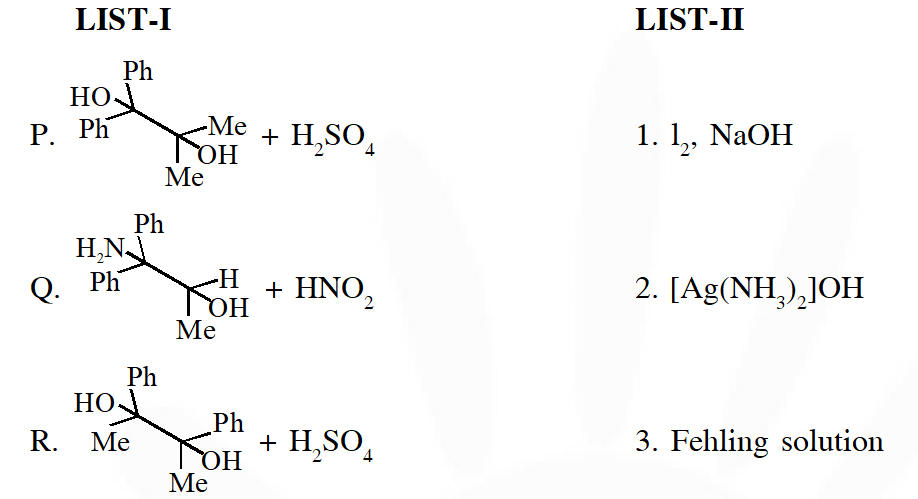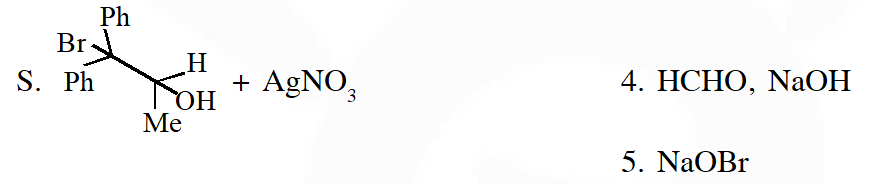The correct option is (A) $\mathrm{P} \rightarrow 1 ; \mathrm{Q} \rightarrow 2,3 ; \mathrm{R} \rightarrow 1,4 ; \mathrm{S} \rightarrow 2,4$ (B) $\mathrm{P} \rightarrow 1,5 ; \mathrm{Q} \rightarrow 3,4 ; \mathrm{R} \rightarrow 4,5 ; \mathrm{S} \rightarrow 3$ (C) $\mathrm{P} \rightarrow 1,5 ; \mathrm{Q} \rightarrow 3,4 ; \mathrm{R} \rightarrow 5 ; \mathrm{S} \rightarrow 2,4$ (D) $\mathrm{P} \rightarrow 1,5 ; \mathrm{Q} \rightarrow 2,3 ; \mathrm{R} \rightarrow 1,5 ; \mathrm{S} \rightarrow 2,3$ JEE Advance 2018
Ans. (D)
Q. LIST-I contains reactions and LIST-II contains major products.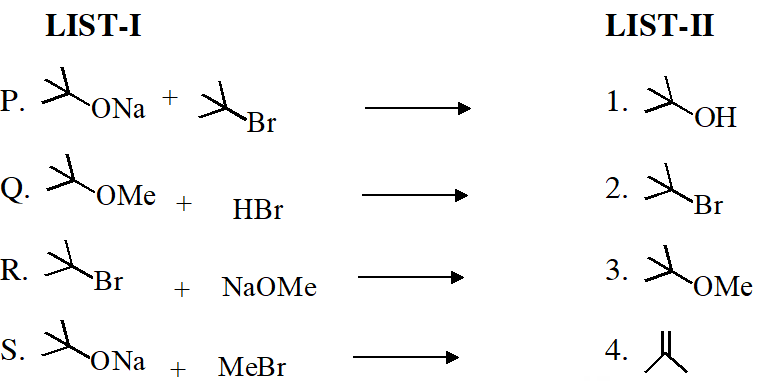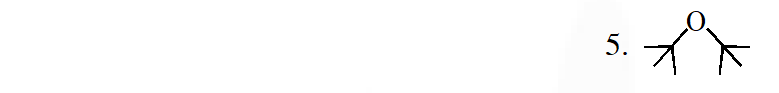Match each reaction in LIST-I with one or more product in LIST-II and choose the correct option. (A) $\mathrm{P} \rightarrow 1,5 ; \mathrm{Q} \rightarrow 2 ; \mathrm{R} \rightarrow 3 ; \mathrm{S} \rightarrow 4$ $(\mathrm{B}) \mathrm{P} \rightarrow 1,4 ; \mathrm{Q} \rightarrow 2 ; \mathrm{R} \rightarrow 4 ; \mathrm{S} \rightarrow 3$ (C) $\mathrm{P} \rightarrow 1,4 ; \mathrm{Q} \rightarrow 1,2 ; \mathrm{R} \rightarrow 3,4 ; \mathrm{S} \rightarrow 4$ (D) $\mathrm{P} \rightarrow 4,5 ; \mathrm{Q} \rightarrow 4 ; \mathrm{R} \rightarrow 4 ; \mathrm{S} \rightarrow 3,4$ JEE Advance 2018
Ans. (B)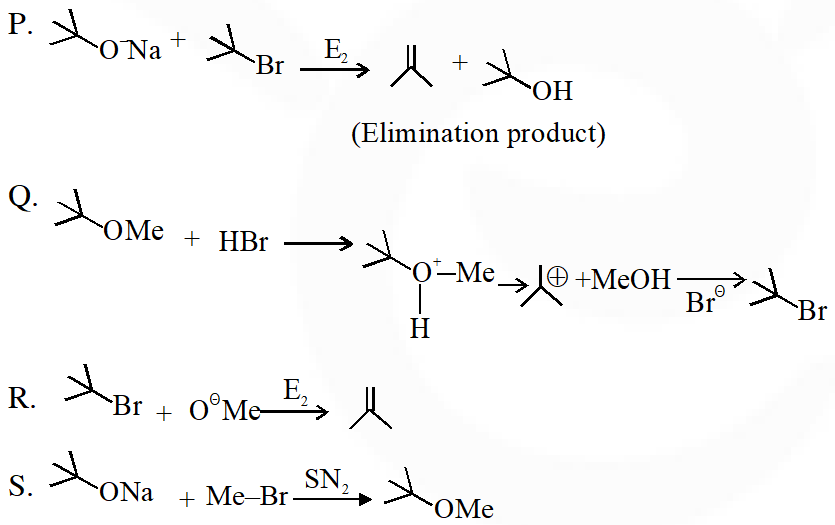M Naga B ushan
Nov. 13, 2020, 7:15 p.m.
nice experience
vartika
Oct. 20, 2020, 1:33 p.m.
2019 ke bhi chahiye
weona
Oct. 20, 2020, 1:27 p.m.
thanks
Pallavi
Oct. 8, 2020, 9:30 a.m.
Yuvraj
Sept. 26, 2020, 6:11 p.m.
Please give 2019 paper also! By the way thank you
Aayush
Sept. 22, 2020, 6:32 p.m.
2019ke kon dega
Gaurav kumar
Sept. 16, 2020, 3:28 p.m.